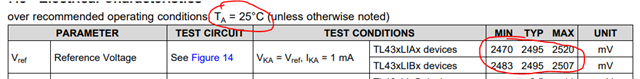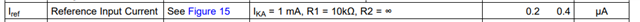If you have a related question, please click the "Ask a related question" button in the top right corner. The newly created question will be automatically linked to this question.

# TL431LI: Deviation of the Vi(dev) and Ii(dev) over full temperature range

Part Number: TL431LI

Hi team,

Could you give me comment on following customer questions?

Q1. Where is the Vref at 25C in the Videv range?

Videv = 11, 17, and 27mV are shown in the datasheet, and the customer understands it is the difference between maximum value and minimum value of the Vref (T).

For example, compared to the Vref (T=25C),

For 0~70C case, +5mV for max value and -6mV for min value, Videv = 11mV

For -40~85C case, +5mV for max value and -12mV for min value, Videv = 17mV,

For -40~125C case, +5mV for max value and -22mV for min value, Videv = 27mV.

Q2. What is the case for Ii(dev)? The customer understands the deviation is 0.3uA, and they want to know where the Iref at 25C is in the Iidev range.

They want to understand like above information for their design calculation.

Best regards,

Takeshi Sasaki

• Hi Takeshi-san

Q1. Where is the Vref at 25C in the Videv range?

VREF is tested at room temp and the range is listed in the datasheet.Q2. What is the case for Ii(dev)? The customer understands the deviation is 0.3uA, and they want to know where the Iref at 25C is in the Iidev range.

Same as Q1, IREF is measured to have a range @25C and is shown in the datasheet.Ben

• Hi Ben,

Thank you for your comment. So, Vref (at 25C) is centered between Vref,min (at 25C) and Vref,max (at 25C). Can the customer calculate Vref,min and Vref,max at given temperature range Q, I, or C like as below?

For example, for TL43xLIAx devices, when temperature range is given as Q,

Vref,max = 2520+13.5mV = 2533.5mV, Vref,min = 2470-13.5mV = 2456.5mV. So It's not reasonable to calculate Vref,max = 2520+5mV, Vref,min = 2470-27mV as the customer mentioned in previous thread. Is it correct understanding?

Can such calculation be applied to Iref and Ii(dev)?

Best regards,

Takeshi Sasaki

• Hi Takeshi-san,

Yes, that is correct and can be applied to the Iref and li(dev).

Ben

• Hi Takeshi-san,

If there are no further questions, please click on "resolved" to close the thread.  Thank you and good luck to you!

Ben